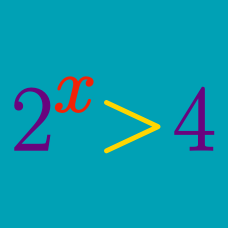Algebra

# Exponential Inequalities - Base < 1

What is the solution set to $\frac{1}{32}\leq\left(\frac{1}{2}\right)^{2x+1} \leq 4?$

What is the solution set to $0.5^{x+4}\geq16?$

If $$0<a<b,$$ the solution set to $a<a^xb^{1-x}<b$ is $$\alpha<x<\beta.$$ Find $$-2\alpha+3\beta.$$

What is the smallest integer $$x$$ that satisfies $\left(\frac{1}{2}\right)^x<16?$

If the solution to $\frac{1}{27}<\left(\frac{1}{9}\right)^x<2187$ is $$\alpha<x<\beta,$$ what is $$\alpha+\beta?$$

×